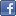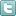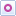EE Times-India > Amplifiers/Converters

Amplifiers/Converters# Performing sine/cosine-to-digital conversion

Posted: 12 Mar 2014Print Version

Keywords:Optical  magnetic sensors  motion control systems  sine/cosine-to-digital conversion  SDC

Optical or magnetic sensors for position and angle sensing in motion control systems usually provide sine and cosine signals for conversion. The 90 phase shift between sine and cosine allows determination of the position or angle within the 360 input cycle, as well as the direction of rotation or movement. For resolutions in the micrometre or sub-arc minute range, precise interpolation of the sine and cosine signals is necessary. This sine/cosine-to-digital conversion (SDC) can be performed in several ways, either in hardware or software. For high-precision results, the quality of the signal conditioning and of the S/D conversion is of major importance. In the following, several SDC (interpolation) methods are analysed and compared, and accuracy results are discussed.

Sensor signal path with S/D conversion
Sensors in electronic position and angle measurement work on optical, magnetic, inductive, or capacitive principles. Optical sensors with LED light source and code disc are very common, as are magnetic GMR/AMR/Hall sensors using a dipole magnet or multi-pole wheel . As shown in figure 1, these sensors usually provide a sine and a cosine signal directly. Because they do not always provide perfect sine/cosine signals, the sensor outputs must be conditioned before interpolation to allow high accuracy results. The goal of this conditioning is to provide—as nearly as is possible—sine/cosine waves with equal amplitude, zero offset, and precisely 90 phase shift. Programmable gain amplifiers (PGAs) and/or lookup tables are often used to provide the required amplitude balancing (gain correction), offset compensation, and phase correction.

Typically, a Z sensor defines the zero position for position/angle measurement and its pulse output is sampled using an adjustable comparator. If no Z sensor is available, the interpolator can define the zero position as well . In this case, the zero position can be set anywhere within the 360 input sine/cosine cycle to generate the Z output at a user-defined position or angle.Figure 1: Signal path for sine/cosine-to-digital conversion.

The interpolator implements the S/D conversion and outputs its result via one of several output interfaces. This digital output can be read directly by a local microcontroller or transmitted via line drivers to a remote control system.

Typical methods of sine/cosine-to-digital conversion
The interpolator is responsible for the non-linear A/D conversion that transforms the sine/cosine signals into position or angle steps (figure 2). These steps are then output either incrementally as quadrature square-wave signals (which include direction information), or as a data word representing the absolute angle within the 360 input cycle.Figure 2: Interpolar signal path.

The non-linear function usually used for Sine/Cosine-to-Digital conversion is the arc tangent, which calculates the output angle directly from the conditioned sine and cosine signals (figure 2). Many different A/D conversion methods can be used to implement the arc tangent function, depending on the application requirements:
• Flash conversion, which uses many individual comparators to perform the conversion almost instantly. Vector-tracking conversion, which uses a single comparator to increment or decrement a digital counter to track the input angle.
• SAR conversion, which is similar to vector-tracking conversion, but which samples and holds the input signal until the counter has settled.
• DSP conversion, which digitizes the sine and cosine signals individually and calculates the arc tangent function in a Digital Signal Processor using a CORDIC or other numerical algorithm.

 Related Articles Editor's Choice
Comment on "Performing sine/cosine-to-digital co..."

Top Ranked Articles

WebinarsVisit Asia Webinars to learn about the latest in technology and get practical design tips.

Search EE Times India
Services

Go to topConnect on FacebookFollow us on TwitterFollow us on Orkut
﻿

Back to Top